Next: Variance of a function Up: Computing the expectation of Previous: Expectation of the description

## Expectation of a function

Equations 6 and 7 show that we could compute the expected description length if we knew how to evaluate the expectations of the functions fi.

Let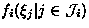be a function whose expectation value we would like to compute. As before, we shall approximate fi with its second order Taylor's series expansion. However, we are not going to expand fi with respect to the discretised parameters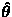of the network but the direct parameters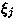of the function fi. The expansion is thus computed about the expectation values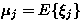.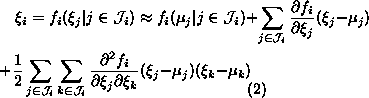Taking expectation from both sides of equation 8 yields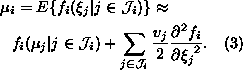Here we have denoted the variance ofby vj: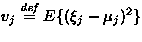. The first order terms disappear because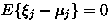. We have also assumed that for all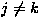, eitherand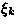are uncorrelated or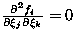, which removes the second order cross terms.

Harri Lappalainen
5/19/1998